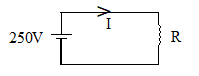In the given circuit, the resistance R has a value that depends on the current in the circuit. R is   when I is zero and the increase in the resistance (in Ω ) is numerically equal to half of the current in ampere. What is the value of I in the circuit?

# In the given circuit, the resistance R has a value that depends on the current in the circuit. R is   when I is zero and the increase in the resistance (in $\mathrm{\Omega }$ ) is numerically equal to half of the current in ampere. What is the value of I in the circuit?1. A

8.33A

2. B

10A

3. C

12.5A

4. D

18.5A

Register to Get Free Mock Test and Study Material

+91

Verify OTP Code (required)